# Higher Order Chemical Reaction on MHD Nanofluid Flow with Slip Boundary Conditions: A Numerical Approach

Higher Order Chemical Reaction on MHD Nanofluid Flow with Slip Boundary Conditions: A Numerical Approach
Department of Mathematics, Radhakrishna Institute of Technology & Engineering, Biju Patnaik University of Technology, Bhubaneswar 752057, India

Department of Mathematics, Institute of Technical Education and Research, Siksha ‘O’ Anusandhan (Deemed to be University), Bhubaneswar 751030, India

Corresponding Author Email:
kharabela1983@gmail.com
Page:
293-299
|
DOI:
https://doi.org/10.18280/mmep.060218
13 August 2018
|
Accepted:
26 February 2019
|
Published:
20 June 2019
| Citation

OPEN ACCESS

Abstract:

The present paper analyzes the MHD flow of nanofluid past a permeable stretched surface. The effect of non-linear radiative heat transfer, higher order chemical reaction and slip boundary conditions are also incorporated to the flow phenomena to enhance the heat transfer rate in the nanofluid. A suitable self-similar transformation is employed to convert PDEs into non-linear ODEs. The resulting set of differential systems is solved numerically by fourth order Runge-Kutta method with shooting technique. The impact of thermo-physical quantities on the flow field is shown via graphs. The numerical results for skin friction coefficient, local Nusselt and Sherwood numbers are calculated and demonstrated via table. It is found that heat generation is favorable to enhance the rate of shear stress as well as rate of heat transfer, further absorption retards mass transfer rate significantly. Also, the thickness of species distribution increases as the order of the chemical reaction n increases.

Keywords:

nanofluid, non-linear thermal radiation, chemical reaction, porous medium

1. Introduction

A nanoparticle is a microscopic particle of diameter less than 100nm. The nanoparticles are made up metals, carbides, oxides or carbon nanotubes. By adding nanoparticles in the convectional fluids like ethylene glycol, water and engine oil, nanofluids are formed to enhance the heat transfer phenomena of the base fluids. There are several applications of nanofluids in the diverse field of engineering such as heat exchanger, refrigerator freezers, coolants, solar receiver, radiators etc. The pioneer experimental work on nanofluid is obtained Choi . In his work, he takes water as a base fluid which enhances the heat transfer properties of the fluid. Further, development of model by incorporating Brownian motion and thermophoresis in the boundary layer flow of nanofluid was studied by Buongiorno .

The heat transfer radiation has many applications in the various fields such as design of engines and combustion chambers to operate at increased temperature to raise thermal efficiency, solar energy, semiconductor wafer processing, manufacturing of translucent crystals, energy transfer in furnaces etc. Akbar et al.  have studied the effects of the thermal radiation on nanofluid flow towards a stretching sheet with convective boundary condition. Second order slip MHD flow with the effects of radiation and chemical reaction has been studied by Zhu et al. . Ibrahim  and Reddy  have considered the MHD nanofluid flow past a stretched surface with convective boundary condition and thermal radiation. Mabood et al.  projected their aim on Williamson nanofluid flow in presence of thermal radiation. Elbashbeshy et al. , Babu and Sandeep , Rahman and Eltayeb  and Mustafa et al.  studied numerically the results of nonlinear radiation mechanism for heat transfer of nanofluid past a vertical plate. Motsumi and Makinde  also extended their work by incorporating viscous dissipation and thermal radiation.

The application of heat and mass transfer is an important aspect now a day in industries such as the application of wet cooling water, drying, curing of plastics, food processing etc. Heat is generated when chemical reaction takes place between nanoparticles and base fluids. At that time the behavior of nanoparticles is observed which depending upon the sign of the chemical reaction. The rate of chemical reaction is controlled by the concentration of the species. Kandasamy and Devi  and Mahantesh et al.  have investigated the mixed convective nanofluid flow past a vertical plate with chemical reaction. Palani et al.  discussed unsteady nanofluid flow phenomena in presence of chemical of higher order.

The aim of the present study is to investigate the boundary layer flow of MHD nanofluid over stretching sheet under the influence of non-linear thermal radiation and higher order chemical reaction. Using similarity transformations, the governing PDEs are transferred into ODEs which were solved numerically by shooting technique. For the validation of the present result we have compared our result with that of earlier established result of Ibrahim  in a particular case.

2. Problem Formalism

Electrically conducting boundary layer flow of nanofluid over a permeable stretched surface immersed in a porous medium as shown in Figure 1 has been considered. Let us assume: (i) the flow is steady (ii) the sheet surface gets heated at temperature T and a heat transfer coefficient hf  (iii) the flow is laminar (iv) B0 , magnetic field strength is applied normal to the flow (v) the induced magnetic field is neglected (vi) no slip boundary conditions are subjected to very thin moving fluid and stationary sheet (vii) the gravitational effect is negligible.

The governing boundary layer equations subjected to the conversation of mass, momentum and energy are respectively

## 1.png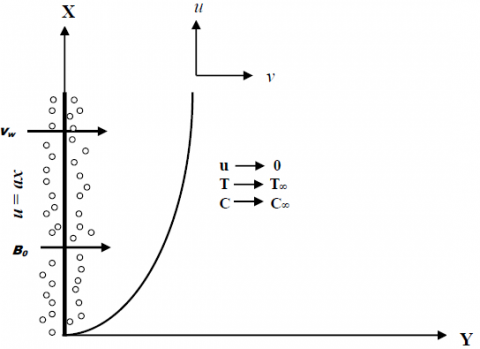Figure 1. Sketch of flow

$\frac{\partial u}{\partial x}+\frac{\partial v}{\partial y}=0$ (1)

$\rho_{f}\left(u \frac{\partial u}{\partial x}+v \frac{\partial u}{\partial y}\right)=\mu_{f} \frac{\partial^{2} u}{\partial y^{2}}-\sigma B_{0}^{2} u-\frac{\mu_{f} u}{K p^{*}}$ (2)

$u \frac{\partial T}{\partial x}+v \frac{\partial T}{\partial y}=\alpha_{f} \frac{\partial^{2} T}{\partial y^{2}}+\frac{(\rho C)_{p}}{(\rho C)_{f}}\left\{D_{B} \frac{\partial C}{\partial y} \frac{\partial T}{\partial y}+\frac{D_{T}}{T_{\infty}}\left(\frac{\partial T}{\partial y}\right)^{2}\right\}$

$-\frac{1}{\left(\rho C_{p}\right)_{f}} \frac{\partial q_{r}}{\partial y}+\frac{\mu_{f}}{\left(\rho C_{p}\right)_{f}}\left(\frac{\partial u}{\partial y}\right)^{2}+\frac{Q_{0}}{\left(\rho C_{p}\right)_{f}}\left(T-T_{\infty}\right)$ (3)

$u \frac{\partial C}{\partial x}+v \frac{\partial C}{\partial y}=D_{B} \frac{\partial^{2} C}{\partial y^{2}}+\frac{D_{T}}{T_{\infty}} \frac{\partial^{2} T}{\partial y^{2}}-K_{n}\left(C-C_{\infty}\right)^{n}$ (4)

Using Rosseland approximation of radiation, the radiative heat flux is given by

$q_{r}=-\frac{4 \sigma^{*}}{3 k^{*}} \frac{\partial T^{4}}{\partial y}=-\frac{16 \sigma^{*}}{3 k^{*}} T^{3} \frac{\partial T}{\partial y}$ (5)

Substituting equation (5) in equation (4) we get

$u \frac{\partial T}{\partial x}+v \frac{\partial T}{\partial y}=\frac{\partial}{\partial y}\left[\left\{\alpha_{f}+\frac{16 \sigma^{*} T^{3}}{3 k^{*}\left(\rho C_{p}\right)_{f}}\right\} \frac{\partial T}{\partial y}\right]$

$+\tau\left\{D_{B} \frac{\partial C}{\partial y} \frac{\partial T}{\partial y}+\frac{D_{T}}{T_{\infty}}\left(\frac{\partial T}{\partial y}\right)^{2}\right\}+\frac{\mu_{f}}{\left(\rho C_{p}\right)_{f}}\left(\frac{\partial u}{\partial y}\right)^{2}$

$+\frac{Q_{0}}{\left(\rho C_{p}\right)_{f}}\left(T-T_{\infty}\right)$ (6)

The surface conditions are:

$u=u_{w}(x)=a x, v=v_{w},-k_{f} \frac{\partial T}{\partial y}=h_{f}\left(T_{f}-T\right)$

$-D_{m} \frac{\partial C}{\partial y}=h_{m}\left(C_{f}-C\right)$ at $y=0$

$u=0, T \rightarrow T_{s}, C \rightarrow C_{p}$ as $y \rightarrow \infty$ (7)

where uw  and vw  are the stretching velocity and wall mass transfer velocity respectively, a>0 for the stretching sheet.

The similarity transformations considered are:

$\eta=\sqrt{\frac{a}{v_{f}}} y, u=a x f^{\prime}(\eta), v=-\sqrt{v_{f} a} f(\eta)$

$\theta(\eta)=\frac{T-T_{\infty}}{T_{f}-T_{\infty}}, \phi(\eta)=\frac{C-C_{\infty}}{C_{f}-C_{\infty}}$$\}$ (8)

Using equation (8), equations (2), (6), and (4) can be written as

$f^{\prime \prime}-f^{\prime 2}+f f^{\prime \prime}-\left(M+\frac{1}{K p}\right) f^{\prime}=0$ (9)

$f^{\prime \prime \prime}-f^{\prime 2}+f f^{\prime \prime}-\left(M+\frac{1}{K p}\right) f^{\prime}=0$ (10)

$\phi^{\prime \prime}+L e \operatorname{Pr} f \phi^{\prime}+\frac{N t}{N b} \theta^{\prime \prime}-\gamma L e \operatorname{Pr} \phi^{n}=0$ (11)

The non-dimensional forms of equation (7) are

$f(0)=S, f^{\prime}(0)=1, \theta^{\prime}(0)=-B i_{t}[1-\theta(0)]$

$\phi^{\prime}(0)=-B i_{c}[1-\phi(0)]$ at $\eta=0$

$f^{\prime}(\infty)=0, \theta(\infty)=0, \phi(\infty)=0$ as $\eta \rightarrow \infty$ (12)

with non-dimensional parameters

$M=\frac{\sigma B_{0}^{2}}{a \rho_{j}}, K p=\frac{a K p^{*}}{v_{f}}, R=\frac{16 \sigma^{*} T_{\infty}^{3}}{3 k_{f} k^{*}}, \operatorname{Pr}=\frac{v_{f}}{\alpha_{f}}$

$N b=\frac{\tau D_{s}\left(C_{f}-C_{\alpha}\right)}{v_{f}}, \gamma=\frac{K_{n}\left(C_{f}-C_{\alpha}\right)^{n-1}}{a}, L e=\frac{\alpha_{f}}{D_{B}}$

$N t=\frac{\tau D_{T}\left(T_{f}-T_{\infty}\right)}{v_{f} T_{\infty}}, E c=\frac{\mu_{f} u_{w}^{2}}{\left(\rho C_{p}\right)_{f}\left(T_{f}-T_{\infty}\right)}, S=\frac{v_{w}}{\sqrt{a v_{f}}}$

$Q=\frac{v_{f} Q_{0}}{a \alpha\left(\rho C_{p}\right)_{f}}, B i_{t}=\frac{h_{f}}{k} \sqrt{\frac{v_{f}}{a}}, B i_{c}=\frac{h_{m}}{D_{m}} \sqrt{\frac{v_{f}}{a}}$

3. Physical Quantities of Engineering Interest

The important physical quantities used in these flow phenomena are shear stress coefficient Cf, local Nusselt number Nux

and local Sherwood number Shx  are defined as

\begin{aligned} C_{f} &=\frac{\tau_{w}}{\rho u_{w}^{2}} \\ N u_{x}=& \frac{x q_{w}}{k_{f}\left(T_{f}-T_{\infty}\right)} \\ S h_{x} &=\frac{x q_{m}}{D_{B}\left(C_{f}-C_{\infty}\right)} \end{aligned} \} (13)

Here, wall shear stress

$\tau_{w}=\mu_{f}\left(\frac{\partial u}{\partial y}\right)_{y=0} \Rightarrow C_{f} \sqrt{\operatorname{Re}_{x}}=-f^{\prime \prime}(0)$

The wall heat flux

$q_{w}=-k\left(\frac{\partial T}{\partial \dot{y}}\right)_{y=0}+\left(q_{r}\right)_{y=0} \Rightarrow \frac{N u_{x}}{\sqrt{\mathrm{Re}_{x}}}=-(1+R) \theta^{\prime}(0)$

The mass flux

$q_{m}=-D_{B}\left(\frac{\partial C}{\partial y}\right)_{y=0} \Rightarrow \frac{S h_{x}}{\sqrt{\mathrm{Re}_{x}}}=-\phi^{\prime}(0)$

where $R e_{x}=\frac{a x^{2}}{v}$ is the local Reynolds number.

4. Results and Discussion

The present study analyzes the boundary layer nanofluid flow past a permeable stretched sheet embedding with porous medium. Influence of thermal radiation and heat generation/absorption has been incorporated in the energy equation. The crux of the present investigation is the higher order solutal concentration. The governing transformed ODEs are nonlinear and coupled those are unable to solve analytically. The roust numerical technique such as Runge-Kutta fourth order method is employed accompanied with shooting to handle these set of ODEs. Initially, the unknown initial conditions for f"(0) , $\theta^{\prime}(0)$  and $\phi^{\prime}(0)$ are chosen and obtained by shooting technique and then solve numerically. The impact of various physical parameters on the flow phenomena are obtained and presented via Figures 2-14 and the computational results of engineering interest are shown in Table 1. The validation of the said results is obtained with that of established results of Ibrahim  in particular cases.

The numerical values of skin friction coefficient, Nusselt number and Sherwood number are calculated for distinct physical parameters such as M, Pr, R, Ec, γ, S, Q, n in Table 1. It is remarked that magnetic parameter (M) as well as mass transfer parameter (S) enhance the skin friction coefficient whereas chemical reaction parameter reduces it. Radiation parameter and chemical reaction parameter increase the heat flux at the surface but adverse effect is observed in case of mass flux. It is seen that Eckert number as well as heat generation/absorption parameter enhance both heat and mass fluxes at the wall. The increasing values of magnetic parameter and mass transfer parameter enhancing the skin friction coefficient and mass flux at the wall whereas opposite effect is noticed in heat flux. It is seen that higher order chemical reaction and Prandtl number has adverse effect on heat flux and mass flux at the wall.

4.1 Velocity distribution

Figure 2 shows the velocity profile for interaction of magnetic parameter (M) and porosity parameter (Kp). It is observed that the velocity reduces with the increase of M. The fact is the inclusion of magnetic field produces a resistive force called Lorentz force which resists the fluid motion throughout the boundary layer. Further, the effect of embedding parameter, the porous matrix is also exhibited. It is clear to note that the presence of porous matrix decelerates the fluid velocity as well. Similar to that of magnetic parameter, porosity also resists the velocity distribution resulted a thicker velocity boundary layer. Figure 3 exhibits the effect S on the velocity distributions. It is seen that increasing values of S decelerates the nanofluid velocity.

## 2.pngFigure 2. Velocity distributions for M and Kp

## 3.png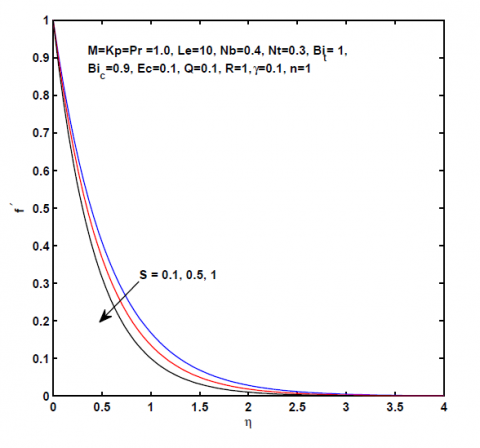Figure 3. Velocity distributions for S

Table 1. Values of skin friction coefficients $f^{\prime \prime}(0),$ Nusselt number $\left\{-\theta^{\prime}(0)\right\}$ and Shervood number $\left\{-\phi^{\prime}(0)\right\}$

 M Pr R Ec $\gamma$ S Q n $f^{\prime \prime}(0)$ $-\theta^{\prime}(0)$ $-\phi^{\prime}(0)$ 0.5 0.7 0.1 0.5 0.1 0.1 0.1 1.0 1.631981 0.074381 0.683601 1.0 1.782788 0.045314 0.687447 1.0 1.782788 0.013137 0.731566 0.5 1.782788 0.054673 0.722572 1.0 1.782788 0.262673 0.757327 -0.1 1.782788 0.264838 0.732148 0.2 -0.1 1.682789 0.322138 0.700694 0 1.732067 0.292476 0.737492 0.1 -0.1 1.782788 0.061230 0.748924 0 1.782788 0.149425 0.755828 0.1 1.782788 0.262129 0.764522 2.0 1.782788 0.263393 0.748703 10.0 1.782788 0.263500 0.747259

4.2 Temperature distribution

Figure 4 illustrates the influences of Prandtl number (Pr) and Lewis number (Le) on the temperature profile. Increase values of Le resulting decrease in temperature profile. Since Pr has inverse relation with thermal conductivity, the larger Pr reverses weaker thermal diffusivity and hence decreases the thermal boundary layer thickness. Figure 5 presents the behavior of the heat transfer for distinct values of Eckert number (Ec). It is seen that an increase in Ec, the heat transfer rate increases. The positive values of Ec indicate cooling of the plate therefore heat loss occurs from the surface to the fluid so that the higher values of dissipative heat energy enhance the fluid temperature. The reason is in the thermal boundary layer certain amount of heat energy stored up which favors in to increase the nanofluid temperature. Figure 6 depicts the importance of thermal radiation on the temperature distribution. It is noteworthy that an increase in R increases the nanofluid temperature. It is the fact that the thermal radiation enhances the thermal diffusivity of the nanofluid resulting a thicker thermal boundary layer. Figure 7 describes the variation of temperature profile for distinct values of heat generation/absorption parameter. It is seen that the heat source parameter enhances the nanofluid temperature whereas the reverse effect is encountered for heat absorption. In the presence of magnetic parameter which retards the velocity profile due to the resistive force at the same time certain amount of heat energy stored up in the thermal boundary layer with an inclusion of heat generation parameter. However, in case of heat absorption due to loss of heat energy the profile becomes thinner and thinner. Figure 8 represents the variation of temperature profile with different values of thermophoresis parameter (Nt) and Brownian motion parameter (Nb). More importantly, the effect of increasing values of both Nt and Nb, increase the temperature profile. In Figure 9, the effects of thermal Biot number ( Bit ) and solutal Biot number ( Bic ) on the temperature distribution is shown. It is note that increasing values of both Bit  and Bic , increase the temperature distribution.

## 4.pngFigure 4. Temperature distributions for Le and Pr

## 5.png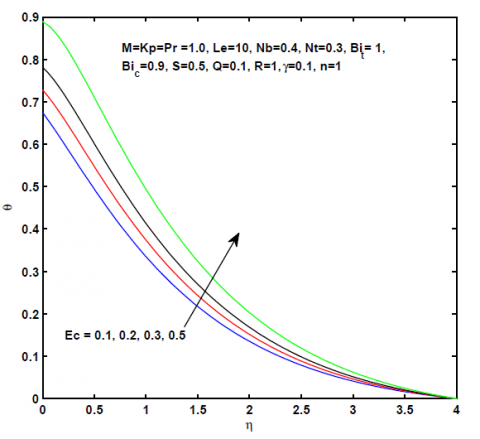Figure 5. Temperature distributions for Ec

## 6.png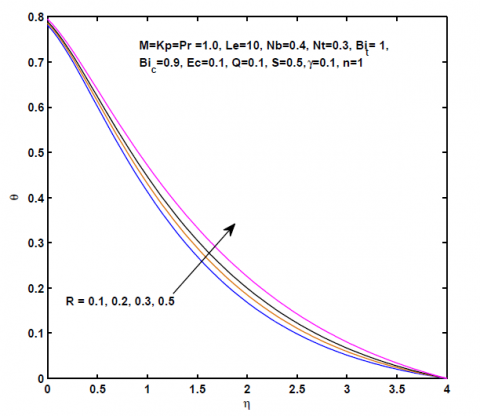Figure 6. Temperature distributions for R

## 7.pngFigure 7. Temperature distributions for Q

## 8.pngFigure 8. Temperature distributions for Nb and Nt

## 9.pngFigure 9. Temperature distributions for and C

4.3 Concentration distribution

The influence of Lewis number (Le) on solutal transfer is demonstrated in Figure 10. As Le is the combined effect of both thermal diffusivity and mass diffusivity, both have retardation effects on the corresponding distribution resulted in the concentration profile decreases. Therefore, as Le increases the solutal boundary layer thickness decreases. Figure 11 displays the effect of $γ$ , chemical reaction parameter on mass transfer distribution. In the present study we consider destructive chemical reaction $γ>0$ , no chemical reaction $γ=0$  and generative chemical reaction $γ<0$ are taken care of. In the absence of chemical reaction present result validates with that of Ibrahim . However, for $γ>0$ , the profiles decelerate and reverse effect is observed for $\gamma <0$ . Figure 12 describes the variation of species concentration for different orders of the chemical reaction. It is remarked that concentration profile enhances with higher order of the chemical reaction. From Figure 13, it is observed that the concentration profile increases as Bic increases. Figure 14 examines the effects of Brownian motion (Nb) and thermophoresis (Nt) parameters on concentration profile. As Nb increases the Brownian force increases for which the concentration gradient of nanoparticles increases at the surface. It is note that higher values of thermophoresis parameter increase the concentration uniformly at all the levels.

5. Conclusions
1. The opposite trend is observed in solutal boundary layer with increasing value of Nb and Nt.
2. Heat generation is favorable to enhance the rate of shear stress as well as rate of heat transfer, further absorption retards mass transfer rate significantly.
3. Thermal boundary layer thickness increases due to increase in both Eckert number Ec and Radiation parameter R.
4. The thickness of species distribution increases as the order of the chemical reaction n increases.
5. The magnitude of concentration $ϕ$  is greater for constructive chemical reaction parameter $γ<0$ in comparison to destructive chemical reaction parameter ( $γ>0$ ).

## 10.png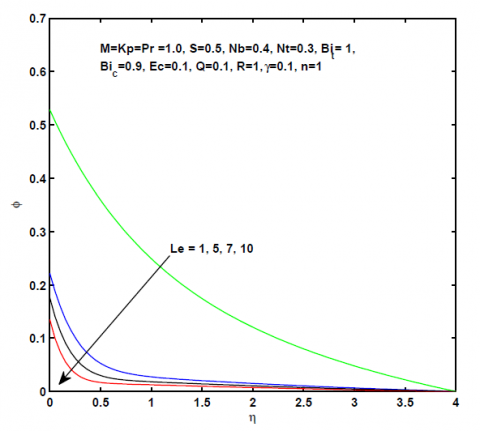Figure 10. Concentration distributions for Le

## 11.pngFigure 11. Concentration distributions for

## 12.pngFigure 12. Concentration distributions for n

## 13.png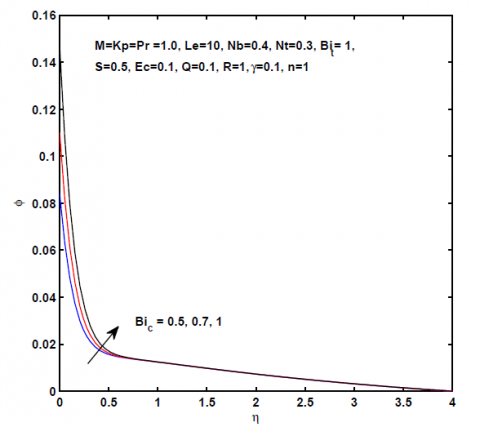Figure 13. Concentration distributions for Bic

## 14.pngFigure 14. Concentration distributions for Nb and Nt

Nomenclature
 u, v Velocity components along the x and y directions respectively M Magnetic parameter Kp Porosity parameter Pr Prandtl number R Thermal radiation parameter Q heat generation/absorption parameter Ec Eckert number S Mass transfer parameter Le Lewis number Nb Brownian motion parameter Nt Thermophoresis parameter $\gamma$ Chemical reaction parameter Bit Thermal Biot number Bic Concentration Biot number ${{\rho }_{f}}$ Density of the fluid ${{\mu }_{f}}$ Dynamic viscosity kf Thermal conductivity of the fluid hf Convective heat transfer coefficient hm Convective mass transfer coefficient Dm Molecular diffusivity $\sigma$ Electrical conductivity $K{{p}^{*}}$ Permeability parameter ${{\alpha }_{f}}$ Thermal diffusivity DB Brownian diffusion coefficient DT Thermophoretic diffusion coefficient T Fluid temperature ${{T}_{\infty }}$ Ambient fluid temperature C Nanoparticle concentration ${{C}_{\infty }}$ Ambient nanoparticle concentration Cp Specific heat at constant pressure Q0 Heat generation/absorption parameter Kn Solutal concentration of order n $\tau =\frac{{{\left( \rho C \right)}_{p}}}{{{\left( \rho C \right)}_{f}}}$ Ratio of effective heat capacity of nanoparticles and heat capacity of the fluid
References

 Choi SUS. (1995). Enhancing thermal conductivity of the fluids with nanoparticles. ASME Int. Mech. Eng. 66: 99-105.

 Buongiorno J. (2006). Convective transport in nanofluids. J. Heat Transfer ASME 128(3): 240-250. http://dx.doi.org/10.1115/1.2150834

 Akbar NS, Nadeem S, Haq RU, Khan ZH. (2013). Radiation effects on MHD stagnation point flow of nanofluid towards a stretching surface with convective boundary condition. Chinese journal of Aeronautics 26(6): 1389-1397. http://dx.doi.org/10.1016/j.cja.2013.10.008

 Zhu J, Zheng L, Zheng L, Zhang X. (2015). Second-order slip MHD flow and heat transfer of nanofluids with thermal radiation and chemical reaction. Appl. Math. Mech. Engl. Ed. 36(9): 1131-1146. http://dx.doi.org/10.1007/s10483-015-1977-6

 Ibrahim W. (2015). Nonlinear radiative heat transfer in magneto hydrodynamic (MHD) stagnation point flow of nanofluid past a stretching sheet with convective boundary condition. Propulsion and Power Research 4(4): 230-239. https://doi.org/10.1016/j.jppr.2015.07.007

 Reddy PBA. (2016). Magnetohydrodynamic flow of a Casson fluid over an exponentially inclined permeable stretching surface with thermal radiation and chemical reaction. Ain Shams Engineering Journal 7: 593-602. http://dx.doi.org/10.1016/j.asej.2015.12.010

 Mabood F, Ibrahim SM, Lorenzini G, Lorenzini E. (2017). Radiation effects on Williamson nanofluid flow over a heated surface with magnetohydrodynamics. International Journal of Heat and Technology 35(1): 196-204. http://dx.doi.org/10.18280/ijht.350126

 Elbashbeshy EMA, Emam TG, Sayed EA. (2016). Effect of thermal radiation on free convection flow and heat transfer over a truncated cone in presence of pressure work and heat generation/absorption. Thermal Science 20(2): 555-565. http://dx.doi.org/10.2298/TSCI130409006E

 Babu MJ, Sandeep N. (2016). Effect of nonlinear thermal radiation on non-aligned bio-convective stagnation point flow of a magnetic-nanofluid over a stretching sheet. Alexandria Engineering Journal 55(3): 1931-1939. https://doi.org/10.1016/j.aej.2016.08.001

 Rahman MM, Eltayeb IA. (2013). Radiative heat transfer in a hydromagnetic nanofluid past a non-linear stretching surface with convective boundary condition. Meccanica 48(3): 601-615. http://dx.doi.org/10.1007/s11012-012-9618-2

 Mustafa M, Mushtaq A, Hayat T, Ahmad B. (2014). Nonlinear radiative heat transfer effect in the natural convective boundary layer flow of a nanofluid past a vertical plate: A numerical study. PLoS ONE 9(9): 1-10. https://doi.org/10.1371/journal.pone.0103946

 Motsumi TG, Makinde OD. (2012). Effects of thermal radiation and viscous dissipation on boundary layer flow of nanofluid over a permeable moving flat plate. Physica Scripta 86(4): 045003. http://dx.doi.org/10.1088/0031-8949/86/04/045003

 Kandasamy R, Devi SPA. (2004). Effects of chemical reaction, Heat and Mass transfer on Nonlinear laminar boundary layer flow over a Wedge with suction or injection. Journal of Computational and Applied Mechanics 5: 21-31. http://dx.doi.org/10.1016/S0735-1933(02)00389-5

 Mahanthesh B, Gireesha BJ, Gorla RSR. (2016). Heat and mass transfer effects on the mixed convective flow of chemically reacting nanofluid past a moving/ stationary vertical plate. Alexandria Engineering Journal 55: 569-581. http://dx.doi.org/10.1016/j.aej.2016.01.022

 Palani S, Rushi K, Kameswaran PK. (2016). Unsteady MHD flow over a stretching surface with higher order chemical reaction. Ain Shams Engineering Journal 7(1): 399-408. http://dx.doi.org/10.1016/j.asej.2015.11.021